﻿ 化学反应速率对爆轰特性的影响研究—基于离散Boltzmann模型 Study on the Influence of Chemical Reaction Rate on Detonation Characteristics—Base on Discrete Boltzmann Model

Vol.04 No.03(2015), Article ID:16220,8 pages
10.12677/CMP.2015.43010

Study on the Influence of Chemical Reaction Rate on Detonation Characteristics

—Base on Discrete Boltzmann Model

Yudong Zhang1, Aiguo Xu2,3,4*, Guangcai Zhang2,4,5, Chengmin Zhu1*

1School of Astronautics, Beijing University of Aeronautics and Astronautics, Beijing

2National Laboratory for Science and Technology on Computational Physics, Institute of Applied Physics and Computational Mathematics, Beijing

3Center for Applied Physics and Technology, MOE Key Center for High Energy Density Physics Simulations, College of Engineering, Peking University, Beijing

4State Key Laboratory of Theoretical Physics, Institute of Theoretical Physics, Chinese Academy of Sciences, Beijing

5State Key Laboratory of Explosion Science and Technology, Beijing Institute of Technology, Beijing

Received: Oct. 8th, 2015; accepted: Oct. 20th, 2015; published: Oct. 26th, 2015ABSTRACT

Based on the high-speed compressible model proposed in our group [Gan, Xu, Zhang, Yang, EPL 103 (2013) 24003], a new discrete Boltzmann model for detonation is presented. A new reaction rate function is adopted which comes from Lee’s model but only the growth term is used. Based on the new model, several kinds of detonations with different reaction rates are simulated and a critical reaction rate is found. In the case where the value of reaction rate equals to the critical value, the simulation results coincide well with CJ theory. In the cases where the reaction rates are lower than the critical value, the von-Neumann peak will appear firstly and then steady state is reached behind the detonation wave. The steady states in those cases are in the CJ detonation states. In the cases where reaction rates are higher than the critical rate, the detonation wave propagates at a speed faster than that of CJ detonation and the steady states in those cases are in the weak detonation states.

Keywords:Discrete Boltzmann Method, Detonation, Reaction Rate Model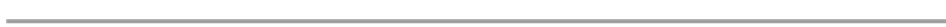—基于离散Boltzmann模型

1北京航空航天大学宇航学院，北京

2北京应用物理与计算数学研究所计算物理重点实验室，北京

3北京大学应用物理与技术研究中心和高能量密度物理数值模拟教育部重点实验室，北京

4理论物理国家重点实验室(中国科学院理论物理研究所)，北京

5爆炸科学与技术国家重点实验室(北京理工大学)，北京1. 引言

2. 模型简介

2.1. DBM模型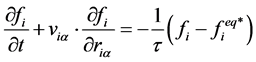(1)

16个离散速度的二维坐标分量为：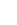2.2. 化学反应率模型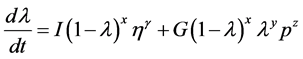(2)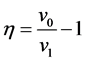(3)Figure 1. Schematic of the discrete velocity model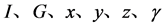均为常数。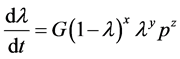(4)(5)

3. 模型验证

3.1. Colella爆炸冲击波问题3.2. 自持稳定爆轰问题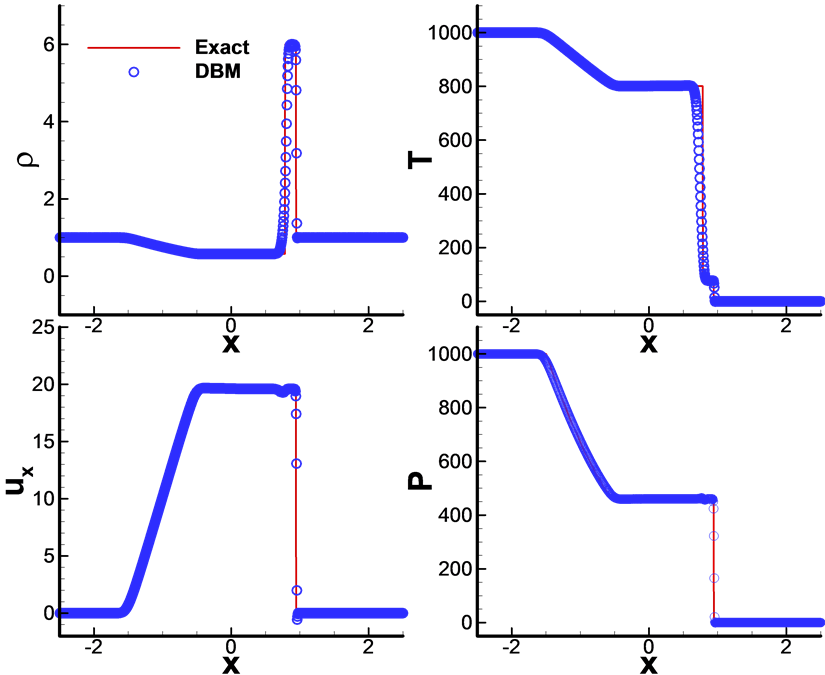Figure 2. Comparisons between DBM result and the exact solutions for Colella’s explosion wave test at the time t = 0.044. 模拟结果及分析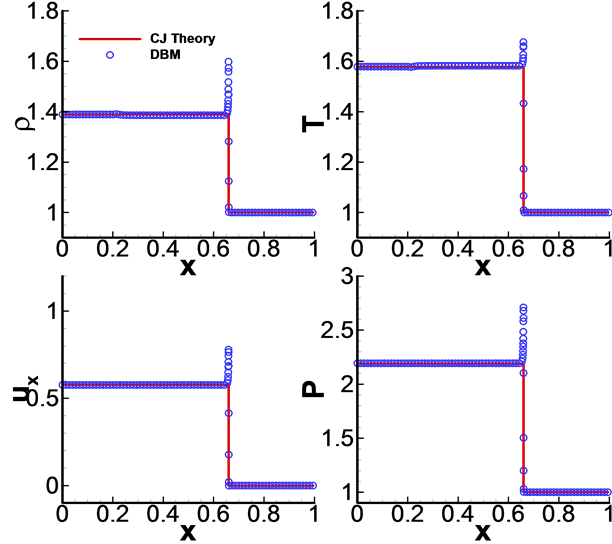Figure 3. Comparisons between DBM result and the CJ theoretical solutions for self sustaining detonation test at the time t = 0.03 (density, temperature, velocity and pressure behind the detonation)Figure 4. Comparisons between DBM result and the CJ theoretical solutions for self sustaining detonation test at the time t = 0.03 (reaction process and the wave velocity)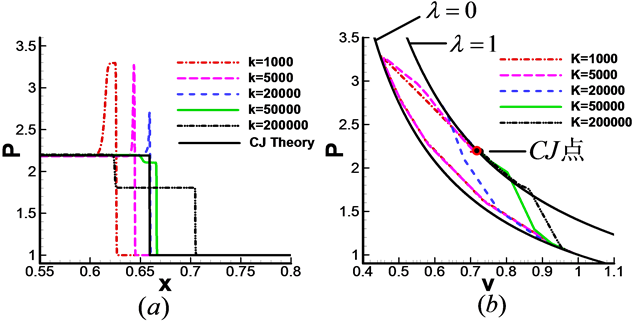Figure 5. Pressure profiles and phase diagram of pressure and specific density at different reaction rates

5. 结论

Study on the Influence of Chemical Reaction Rate on Detonation Characteristics—Base on Discrete Boltzmann Model[J]. 凝聚态物理学进展, 2015, 04(03): 85-92. http://dx.doi.org/10.12677/CMP.2015.43010

1. 1. 姜宗林, 滕宏辉, 刘云峰 (2012) 气相爆轰物理的若干研究进展. 力学进展, 2, 129-140.

2. 2. 孙锦山, 朱建士 (1995) 理论爆轰物理. 国防工业出版社, 北京.

3. 3. Nikolaev, Yu.A., Vasil’ev, A.A. and Ul’yanitskii, B.Yu. (2003) Gas detonation and its application in engineering and technologies. Combustion, Explosion, and Shock Waves, 39, 382-410. http://dx.doi.org/10.1023/A:1024726619703

4. 4. Ju, Y.G. (2014) Recent progress and challenges in fundamental combustion research. Advances in Mechanics, 44, 1-72.

5. 5. Mader, C.L. (1979). Numerical modeling of detonations. University of California Press, Berkeley.

6. 6. Succi, S. (2001) The lattice Boltzmann equation for fluid dynamics and beyond. Oxford University, New York.

7. 7. 郭照立, 郑楚光 (2008) 格子Boltzmann方法的原理及应用. 科学出版社, 北京.

8. 8. 何雅玲, 王勇, 李庆 (2008) 格子Botlzmann方法的理论及应用. 科学出版社, 北京.

9. 9. Xu, A.G., Zhang, G.C., Gan, Y.B., et al. (2012) Lattice Boltzmann modeling and simulation of compressible flows. Frontiers of Physics, 7, 582-560. http://dx.doi.org/10.1007/s11467-012-0269-5

10. 10. 许爱国, 张广财, 李英骏, 李华 (2014) 非平衡与多相复杂系统模拟研究—Lattice Boltzmann 动理学理论与应用. 物理学进展, 3, 136-167.

11. 11. 许爱国, 张广财, 应阳君 (2015) 燃烧系统的离散Boltzmann建模与模拟研究. 物理学报, 18, 1-26.

12. 12. 林传栋, 许爱国, 张广财, 李英骏 (2013) 使用极坐标格子玻尔兹曼方法研究冲击过程–复杂系统中非平衡效应的探索. 凝聚态物理学进展, 2, 88-96.

13. 13. Xu, A.G., Lin, C.D., Zhang, G.C., et al. (2015) Multiple-relaxation-time lattice Boltzmann kinetic model for combustion. Physical Review E, 91, 1-16. http://dx.doi.org/10.1103/PhysRevE.91.043306

14. 14. Gan, Y.B., Xu, A.G., Zhang, G.C., et al. (2013) Lattice BGK kinetic model for high-speed compressible flows: Hydrodynamic and nonequilibrium behaviors. Europhysics Letter, 103, p1-p6. http://dx.doi.org/10.1209/0295-5075/103/24003

15. 15. Gan, Y.B., Xu, A.G., Zhang, G.C., et al. (2015) Discrete Boltzmann modeling of multiphase flows: Hydrodynamic and thermodynamic non-equilibrium effects. Soft Matter, 11, 5336-5345. http://dx.doi.org/10.1039/C5SM01125F

16. 16. Yan, B., Xu, A.G., Zhang, G.C., et al. (2013) Lattice Boltzmann model for combustion and detonation. Frontiers of Physics, 8, 94-110. http://dx.doi.org/10.1007/s11467-013-0286-z

17. 17. Lin, C.D., Xu, A.G., Zhang, G.C., et al. (2014) Polar coordinate lattice Boltzmann kinetic modeling of detonation phenomena. Communications in Theoretical Physics, 62, 737-748. http://dx.doi.org/10.1088/0253-6102/62/5/18

18. 18. 曹威, 何中其, 陈网桦 (2014) TNT后燃反应的水下爆炸实验研究与数值模拟. 高压物理学报, 4, 443-119.

19. 19. 许越 (2004) 化学反应动力学. 化学工业出版社, 北京.

20. 20. Laidler, K.J. (1987) Chemical kinetics. 3rd Edition, Harper & Row, New York.

21. 21. 水鸿寿 (1989) 一维流体力学差分方法. 国防工业出版社, 北京.